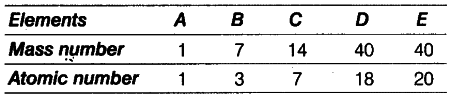# How many neutrons are present in B and D?

In the following table, the mass number and the atomic number of certain elements are given.(i) How many neutrons are present in B and D?
(ii) What would be the valency of element ‘B’ listed in the above table?

(i) Number of neutrons in B=7 -3 = 4
Number of neutrons in D= 40 -18 = 22
(ii) Electronic configuration of the element B is 2, 1. Therefore, its valency is 1.